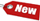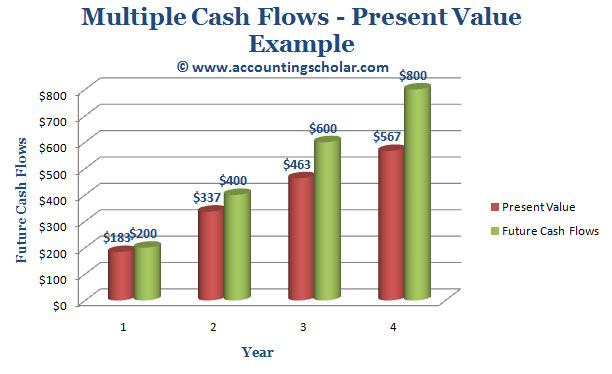Browse Accounting Lessons HereAccounting Terms & DefinitionsAccounting for Merchandising ActivitiesDebits and Credits (Double Entry Accounting)Business Valuation FormulasTime Value of Money & Present/Future ValuesComplex Debt & Equity InstrumentsCommon Stock & Shareholder's EquityAccounting & Finance RatiosValuing Common StockCorporate Income TaxesLower of Cost or Market (LCM) & Inventory ValuationChart of Accounts & BookkeepingBonds Payable & Long Term LiabilitiesCapital AssetsGAAP, Accrual & Cash Accounting, Information Commodity, Internal Controls & MaterialityWhat category of browser are you on this website? Accounting student (homework help) Finance professor (university research) Accounting manager (at work) Other Explore Careers in Accounting and FinanceVisit our section on Careers in Accounting & Finance to explore vast opportunities in this industry.

Chapter 4.13® - Determining Present Value of Multiple Future Cash Flows – Homework Example

Question:

Suppose you are offered an investment that will pay you \$200 in one year, \$400 the next year, \$600 the following year and \$800 at the end of next year. You can earn 9% on such similar investments. That being said, what is the most you would be willing to pay for this investment? In other words, what is the present value of this investment?

Solution:

To solve this problem, we need to calculate the present value of each of these cash flows at 9%. Let’s deal with each one, one at a time:
Note: To solve this problem, we must use the present value discount factor explained in the time value of money chapter.

 Present Value Discount Factor = \$1 x [1/ (1 + r) t] = \$1/ (1 + r) t \$200 x 1 / 1.091 = \$183.49 \$400 x 1 / 1.092 = \$336.67 \$600 x 1 / 1.093 = \$463.31 \$800 x 1 / 1.094 = \$566.74 Total = \$183.49 + \$336.67 + \$463.31 + \$566.74 Total = \$1,550.21

Below is the data summarized in tabular format and following it is the data shown on a pretty little graph:

 Year Present Value Future Cash Flows 1 \$183 \$200 2 \$337 \$400 3 \$463 \$600 4 \$567 \$800 Total: \$1,550 \$2,000

Notice from this graph that for instance if at the end of Year 1 we receive a payment of \$200 as cash flows, then the present value or discounted value of that cash flow at the end of the year is \$183.49. Similarly, an investment that pays \$400 at the end of the 2nd year is discounted for present value at \$337 now. We can perform these calculations using our financial calculator, let’s actually do it to compare our results.This graph shows the breakdown of the annual cash payments of \$200 in the first year, \$400 in the 2nd year, \$600 in the third and so on and breaks down the present values of each of these cash flows in a graphical format.

i) Find Present Value of \$200 at Year 1, 9% rate of return.

 N = 1 I/Y = 9% PV = ? PMT = \$0 FV = \$200 2ND I/Y P/Y = 1 C/Y = 1 CPT & PV = \$183.49

ii) Find Present Value of \$400 at Year 2, 9% rate of return.

 N = 2 I/Y = 9% PV = ? PMT = \$0 FV = \$400 2ND I/Y P/Y = 1 C/Y = 1 CPT & PV = \$336.67

iii) Find Present Value of \$600 at Year 3, 9% rate of return.

 N = 3 I/Y = 9% PV = ? PMT = \$0 FV = \$600 2ND I/Y P/Y = 1 C/Y = 1 CPT & PV = \$463.31

iv) Find Present Value of \$800 at Year 4, 9% rate of return.

 N = 4 I/Y = 9% PV = ? PMT = \$0 FV = \$800 2ND I/Y P/Y = 1 C/Y = 1 CPT & PV = \$566.74